﻿ 基于小波分解与GA-LSSVM的GPS可降水量短临预报文章快速检索 高级检索
 大地测量与地球动力学2019, Vol. 39Issue (5): 487-491  DOI: 10.14075/j.jgg.2019.05.009### 引用本文XIE Shaofeng, SU Yongning, LIU Chunli, et al. Short-Impending Prediction of GPS Precipitable Water Vapor Based on Wavelet Decomposition and GA-LSSVM[J]. Journal of Geodesy and Geodynamics, 2019, 39(5): 487-491.### Foundation support

National Natural Science Foundation of China, No. 41864002, 41704027; Guangxi Natural Science Foundation, No.2018GXNSFAA281182, 2017GXNSFBA198139; Fund of Guangxi Key Laboratory of Spatial Information and Geomatics, No. 15-140-07-11.

### About the first author

XIE Shaofeng, professor, majors in GNSS meteorology, E-mail:xieshaofeng111@126.com.

### 文章历史

1. 桂林理工大学测绘地理信息学院，桂林市雁山街319号，541006;
2. 广西空间信息与测绘重点实验室，桂林市雁山街319号，541006

1 算法原理 1.1 小波分解

 \left.\begin{aligned} c_{j+1} &=H c_{j} \\ d_{j+1} &=G d_{j} \end{aligned}\right\} (1)

 $C_{j}=H^{*} C_{j+1}+G^{*} D_{j+1}$ (2)

cJd1d2、…、dJ分别进行重构，重构后的信号为CJD1D2、…、DJ，则：

 $X=C_{J}+D_{1}+D_{2}+\cdots+D_{J}$ (3)

1.2 最小二乘支持向量机

 $f(\boldsymbol{x})=\boldsymbol{\omega}^{\mathrm{T}} \varphi(\boldsymbol{x})+b$ (4)

 $\min\limits_{\omega, b, e} J(\boldsymbol{\omega}, e)=\boldsymbol{\omega}^{\mathrm{T}} \boldsymbol{\omega}+\frac{1}{2} \gamma \sum\limits_{k=1}^{N} e_{k}^{2}$ (5)
 $y_{k}=\boldsymbol{\omega}^{\mathrm{T}} \varphi\left(x_{k}\right)+b+e_{k}, k=1, \cdots, N$ (6)

 $\begin{array}{c}{L(\boldsymbol{\omega}, b, e, \boldsymbol{a})=J(\boldsymbol{\omega}, e)-} \\ {\sum\limits_{k=1}^{N} a_{k}\left\{\boldsymbol{\omega}^{\mathrm{T}} \varphi\left(x_{k}\right)+b+e_{k}-y_{k}\right\}}\end{array}$ (7)

 $\left[ \begin{array}{cc}{0} & {\boldsymbol{E}_{v}^{\mathrm{T}}} \\ {\boldsymbol{E}_{v}^{\mathrm{T}}} & {\mathit{\boldsymbol{ \boldsymbol{\varOmega}}}+\frac{1}{\gamma} \boldsymbol{I}}\end{array}\right] \left[ \begin{array}{l}{b} \\ {\boldsymbol{a}}\end{array}\right]=\left[ \begin{array}{l}{0} \\ {\boldsymbol{y}}\end{array}\right]$ (8)

 $y(x)=\sum\limits_{k=1}^{N} a_{k} k\left(x, x_{k}\right)+b$ (9)

 $k\left(x_{k}, x_{l}\right)=\exp \left(\frac{-\left\|x_{k}-x_{l}\right\|}{2 \sigma^{2}}\right)$ (10)

σγ对LSSVM的泛化性能至关重要。实际应用中通常采用试凑法或凭经验确定，不但耗时耗力，而且效果还可能不好。所以，本文利用遗传算法的全局寻优能力来确定σγ

1.3 遗传算法

1) 输入训练数据。

2) 种群初始化。个体编码方法采用实数编码，对LSSVM的σ2γ进行编码。

3) 计算个体的适应度值。计算适应度目标函数，目的是寻找一组参数(γ, σ2)，通过LSSVM训练使得式(11)目标函数全局最小，也就是适应度值最大。然后判断是否满足精度要求，若满足则执行步骤7)，否则执行步骤4)。

 $\left\{\begin{array}{l}{\min f\left(\gamma, \sigma^{2}\right)=\frac{1}{2 n} \sum\limits_{i=1}^{n}\left(y_{i}-\hat{y}_{i}\right)^{2}} \\ {\text { s.t }~~ \gamma \in\left[\gamma_{\min }, \gamma_{\max }\right], \quad \sigma^{2} \in\left[\sigma_{\min }^{2}, \sigma_{\max }^{2}\right]}\end{array}\right.$ (11)

4) 选择。筛选适应度优良的个体遗传给下一代，以提高全局收敛性和计算速率。

5) 交叉。随机选择第k个染色体αk和第l个染色体αlj位的基因进行交换，产生新的优秀个体：

 \left.\begin{aligned} \alpha_{k j} &=\alpha_{k j}(1-\beta)+\alpha_{l j} \beta \\ \alpha_{l j} &=\alpha_{l j}(1-\beta)+\alpha_{k j} \beta \end{aligned}\right\} (12)

6) 变异。从种群中任意选取第i个个体的第j个染色体变异为更加优秀的个体：

 $\alpha_{i j}=\left\{\begin{array}{l}{\alpha_{i j}+\left(\alpha_{i j}-\alpha_{\max }\right) f(g), r \geqslant 0.5} \\ {\alpha_{i j}+\left(\alpha_{\min }-\alpha_{i j}\right) f(g), r<0.5}\end{array}\right.$ (13)

7) 终止循环，得到最佳染色体。将遗传算法优化得到的最优个体分解为σ2γ，用于预报。

2 基于WD-GA-LSSVM的可降水量短临预报

1) 把GPS可降水量的原始时间序列x(t)通过小波分解得到1个近似分量cji个细节分量d1d2、…、di

2) 对分解后的各分量分别进行LSSVM建模，并利用遗传算法优化其参数，得到各分量的预报值。本文设置遗传算法的参数为：迭代次数100，种群规模20，交叉概率0.9，变异概率0.2。

3) 对各分量的预报值进行叠加重构，得到可降水量的最终预报结果。

3 实验分析图 1 年积日186 d时间序列及其小波分解结果 Fig. 1 The time series of doy 186 and its decomposition results表 1 年积日186 d各分量参数设置 Tab. 1 Parameter settings of each component for doy 186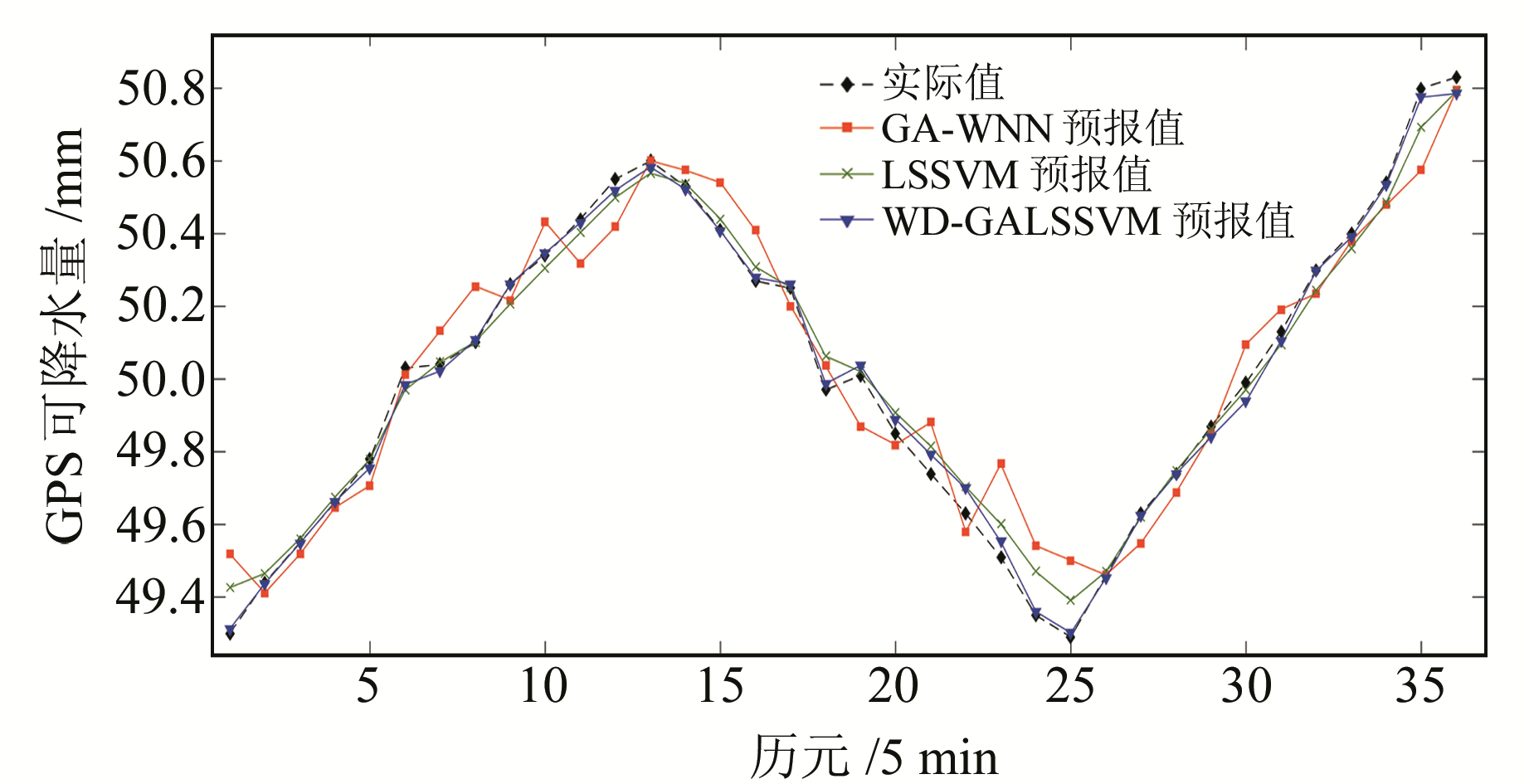图 2 年积日186 d各模型预报值和实际值对比 Fig. 2 Comparison between the predicted values of each model and the actual value for doy 186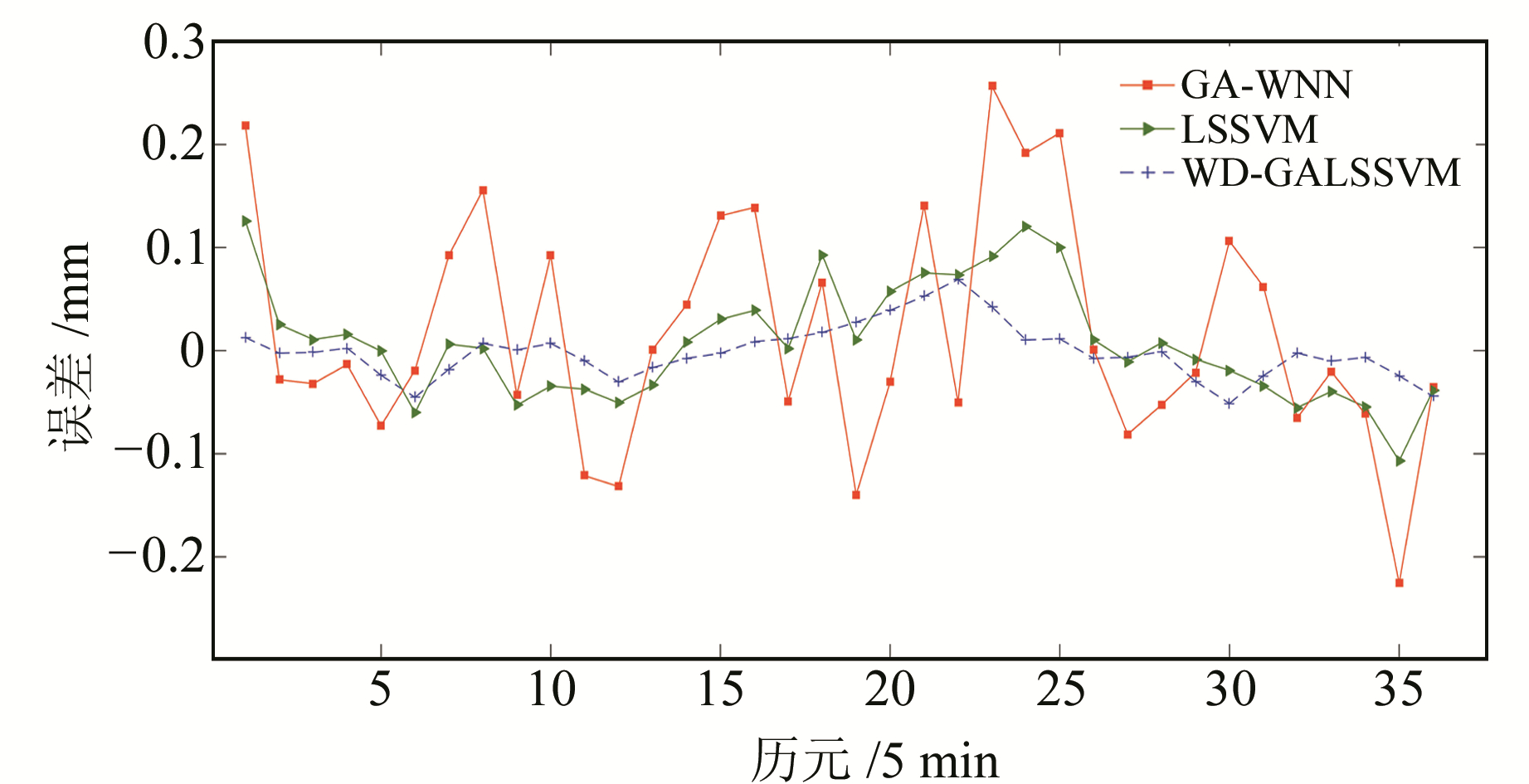图 3 年积日186 d各模型残差 Fig. 3 Residual of each model for doy 186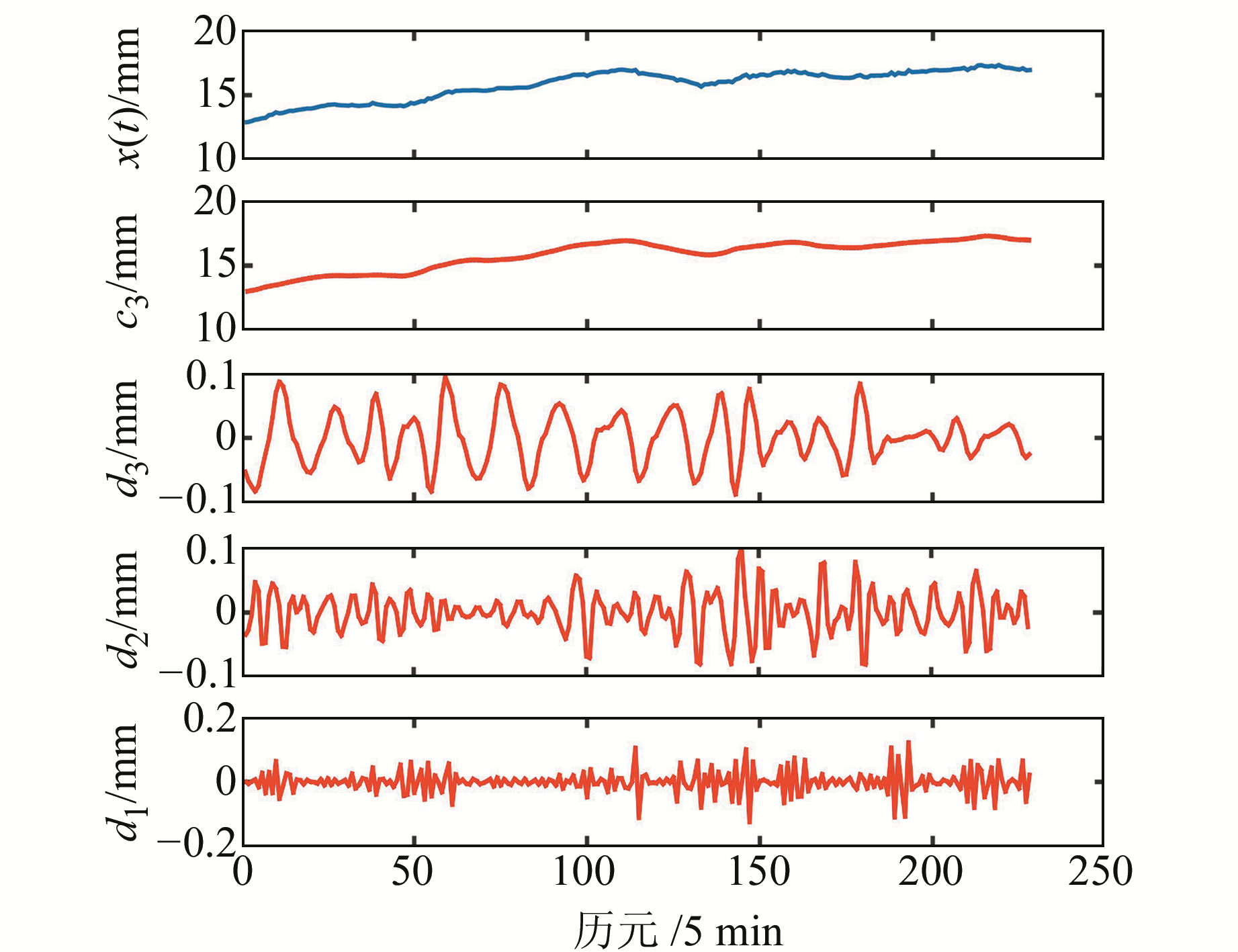图 4 年积日325 d时间序列及其小波分解结果 Fig. 4 The time series of doy 325 and its decomposition results表 2 年积日325 d各分量参数设置 Tab. 2 Parameter settings of each component for doy 325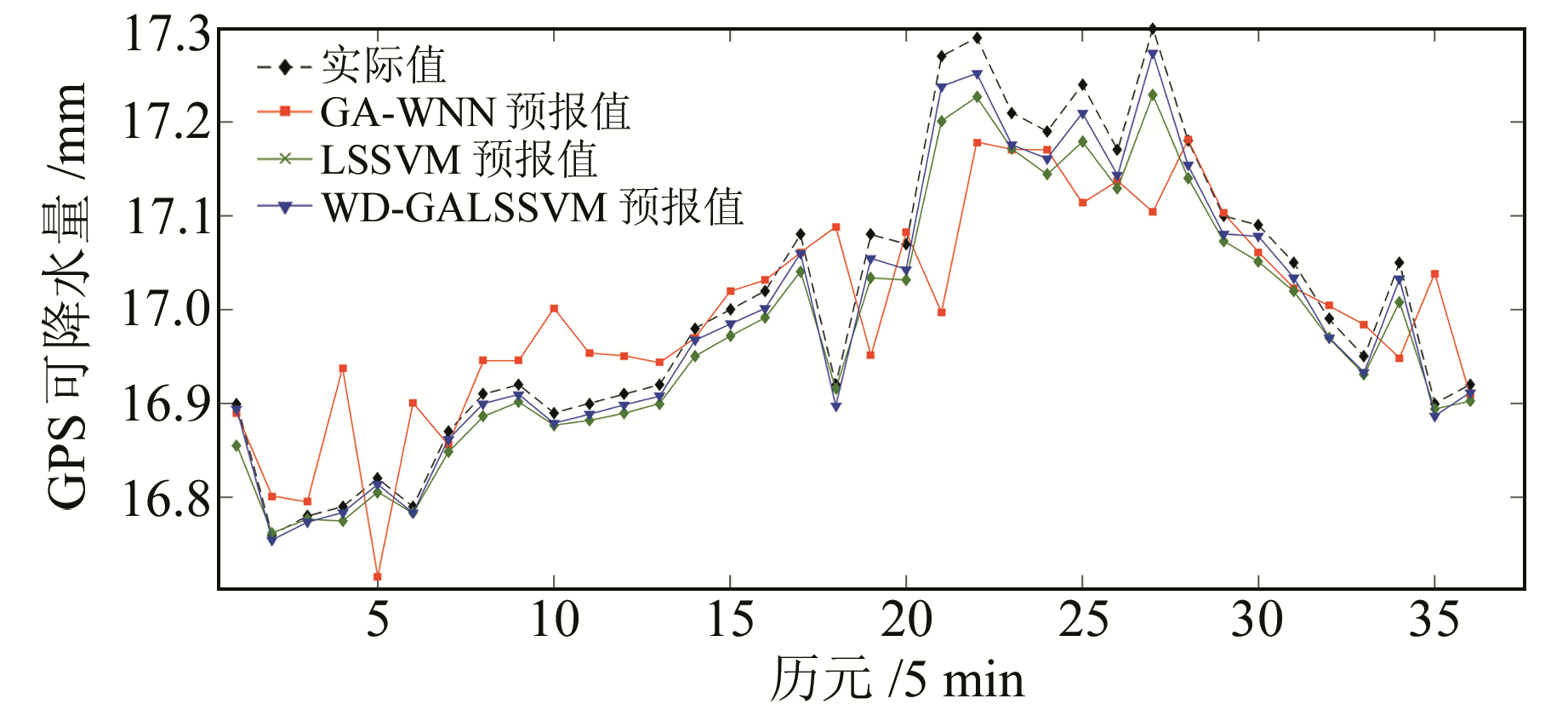图 5 年积日325 d各模型预报值和实际值对比 Fig. 5 Comparison between the predicted values of each model and the actual value for doy 325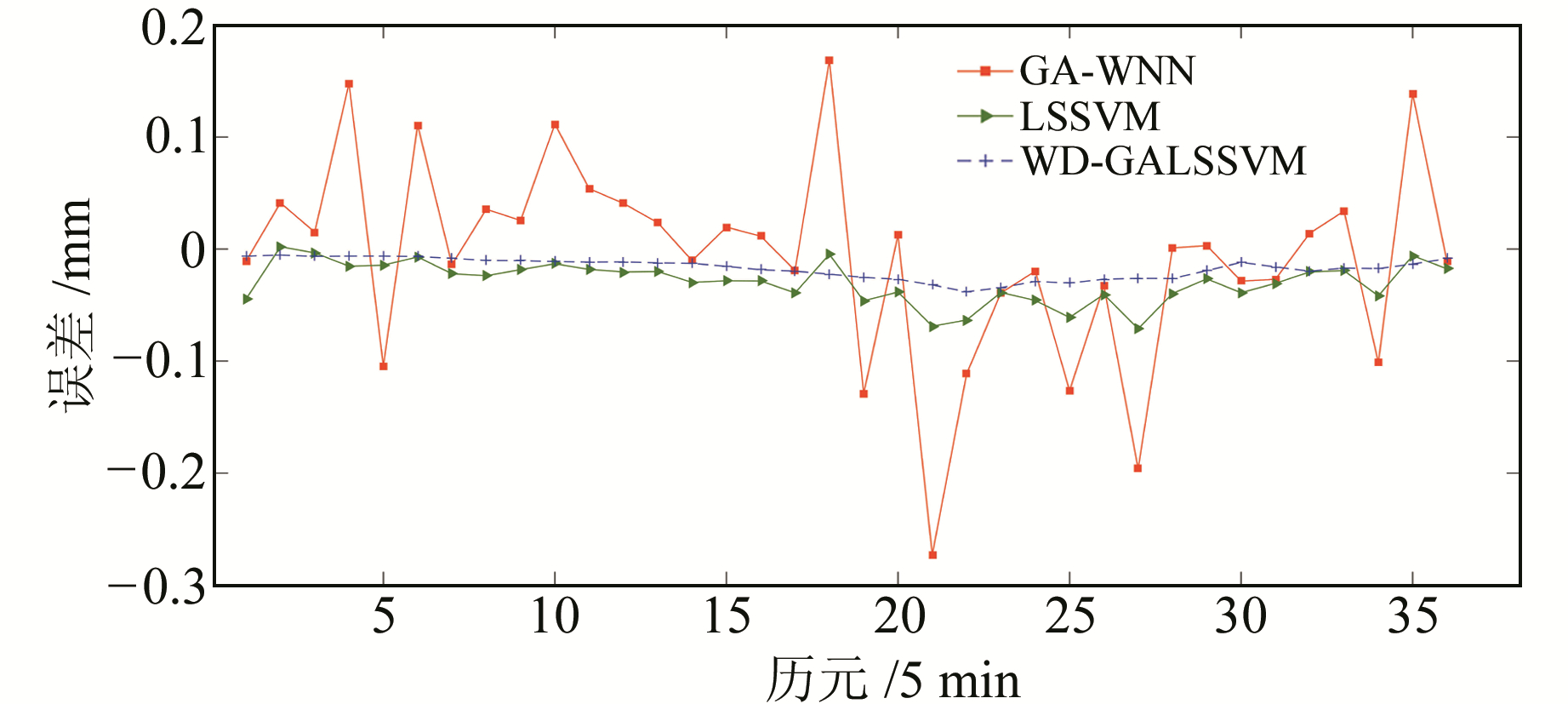图 6 年积日325 d各模型残差 Fig. 6 Residual of each model for doy 325表 3 各模型精度对比 Tab. 3 Comparison of the accuracy of each model

4 结语

  刘严萍, 杨晶, 王勇. 经验模态分解与神经网络的GPS可降水量预测[J]. 测绘科学, 2013, 38(5): 91-93 (Liu Yanping, Yang Jing, Wang Yong. Prediction of GPS Water Vapor Based on EMD and ANN[J]. Science of Surveying and Mapping, 2013, 38(5): 91-93) (0)  张朝菡.基于EMD与RBF的GPS可降水量的降水预报研究[D].唐山: 河北联合大学, 2015 (Zhang Zhaohan. Research on Precipitation Forecast of GPS Precipitable Water Vapor Based on EMD and RBF[D]. Tangshan: Hebei United University, 2015) http://cdmd.cnki.com.cn/Article/CDMD-10081-1015808436.htm (0)  葛玉辉, 熊永良, 陈志胜, 等. 基于小波神经网络的GPS可降水量预测研究[J]. 测绘科学, 2015, 40(9): 28-32 (Ge Yuhui, Xiong Yongliang, Chen Zhisheng, et al. Prediction Method of GPS Precipitation Based on Wavelet Neural Network[J]. Science of Surveying and Mapping, 2015, 40(9): 28-32) (0)  王建敏, 黄佳鹏, 席克伟, 等. 大气可降水量预测模型的自适应Kalmam滤波改进[J]. 测绘科学, 2017, 42(12): 127-133 (Wang Jianmin, Huang Jiapeng, Xi Kewei, et al. Improved Prediction Model of Precipitable Water Vapor Using Adaptive Kalman Filter[J]. Science of Surveying and Mapping, 2017, 42(12): 127-133) (0)  杨锡运, 孙宝君, 张新房, 等. 基于相似数据的支持向量机短期风速预测仿真研究[J]. 中国电机工程学报, 2012, 32(4): 35-41 (Yang Xiyun, Sun Baojun, Zhang Xinfang, et al. Short-Term Wind Speed Forecasting Based on Support Vector Machine with Similar Data[J]. Proceedings of the CSEE, 2012, 32(4): 35-41) (0)  王晓兰, 王明伟. 基于小波分解和最小二乘支持向量机的短期风速预测[J]. 电网技术, 2010, 34(1): 179-184 (Wang Xiaolan, Wang Mingwei. Short-Term Wind Speed Forecasting Based on Wavelet Decomposition[J]. Power System Technology, 2010, 34(1): 179-184) (0)  朱家元, 陈开陶, 张恒喜. 最小二乘支持向量机算法研究[J]. 计算机科学, 2003, 30(7): 157-159 (Zhu Jiayuan, Chen Kaitao, Zhang Hengxi. Study of Least Squares Support Vector Machines[J]. Computer Science, 2003, 30(7): 157-159 DOI:10.3969/j.issn.1002-137X.2003.07.044) (0)  阎威武, 邵惠鹤. 支持向量机和最小二乘支持向量机的比较及应用研究[J]. 控制与决策, 2003, 18(3): 358-360 (Yan Weiwu, Shao Huihe. Application of Support Vector Machines and Least Squares Support Vector Machines to Heart Disease Diagnoses[J]. Control and Decision, 2003, 18(3): 358-360 DOI:10.3321/j.issn:1001-0920.2003.03.025) (0)  鲁凤, 徐建华, 王占永. 合肥市需水预测遗传小波神经网络模型研究[J]. 测绘科学, 2013, 38(5): 28-31 (Lu Feng, Xu Jianhua, Wang Zhanyong. Model of Genetic Algorithms Optimized Wavelet Neural Network on Urban Water Demand Prediction of Hefei[J]. Science of Surveying and Mapping, 2013, 38(5): 28-31) (0)  王小川. MATLAB神经网络43个案例分析[M]. 北京: 北京航空航天大学出版社, 2013 (Wang Xiaochuan. Analysis of 43 Cases of MATLAB Neural Network[M]. Beijing: Beihang University Press, 2013) (0)
Short-Impending Prediction of GPS Precipitable Water Vapor Based on Wavelet Decomposition and GA-LSSVM
XIE Shaofeng1,2SU Yongning1     LIU Chunli1     LIU Lilong1,2
1. College of Geomatics and Geoinformation, Guilin University of Technology, 319 Yanshan Street, Guilin 541006, China;
2. Guangxi Key Laboratory of Spatial Information and Geomatics, 319 Yanshan Street, Guilin 541006, China
Abstract: Aiming at the random and nonlinear characteristic of the time series of GPS precipitable water vapor(PWV), this paper proposes a new short-impending prediction method of GPS PWV based on wavelet decomposition(WD), genetic algorithm(GA) and least squares support vector machine(LSSVM). First, WD is used to decompose the GPS PWV time series into low frequency and high frequency components, which are easy to predict. Second, GA is used to optimize the parameters of LSSVM, and the prediction model of each component is established. Finally, the results of each component prediction are superimposed and reconstructed to get the final prediction results. In this paper, two groups of data are selected for experiments, and the prediction results are compared with those of LSSVM and genetic wavelet neural network(GA-WNN). The results show that the combined model has good generalization ability, can effectively solve the problem that neural network tends to trap in local minimum, and improves global prediction accuracy.
Key words: GPS precipitable water vapor; wavelet decomposition; genetic algorithm; least squares support vector machine; short-impending prediction